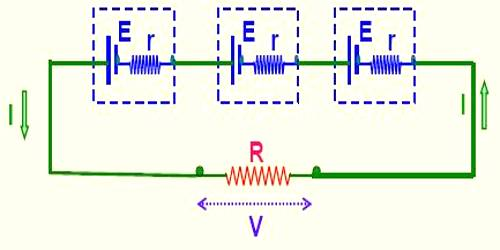Physics

# Series Combination of a CellSeries combination: If some electric cells are connected in such a way as the negative plate of the first cell is connected to the positive plate of the second, negative plate of the second is connected to the positive plate of the third and so on, and then this combination is called series combination. Cells employ chemical reaction to produce an electrical potential. Series cells are produced by individual cells connected together.Suppose that ‘n’ numbers of electric cells are connected with the two ends of a resistor of value R [Figure]. The electromotive force and internal resistance of each cell are respectively E and r. The total electromotive force of the cells or the electromotive force of the battery or potential difference of the circuit is nE and the equivalent internal resistance is nr. This is because that the resistances of the cells are connected in series. Again internal resistance of the cells is connected in series with R. So, the total resistance of the circuit = nr + R.

According to Ohm’s law, current flowing in the circuit

iS = [total electromotive force / total resistance]

= nE / (nr + R)

(1) If R is greater than ‘nr’ i.e., nr ˂ R, then ‘nr’ can be ignored. In this condition, iS = nE/R = n x current produced by a single cell > nE/(nr + R).

So, in order to create a current of large value cells are connected in such a way as R becomes very large compared to ‘nr’.

(2) If R is smaller than nr, then R can be ignored and we get, iS = nE/nr = E/r = current produced by a single cell.

In this situation, the efficiency of the battery does not increase.